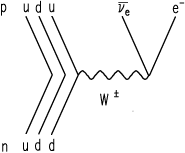# Introduction to Neutron Decay

Home  |   Neutron Decay  |   Perkeo III  |   Perkeo II  |   The Team  |   Publications  |   Links

We examine the fundamentals of the electroweak interaction using cold, polarized neutrons. These decay in an electron, a proton and an anti-electronneutrino with a mean lifetime of about 15 minutes.

In comparison to the beta-decay of nuclei, no disturbing nuclear effects which are based on nuclear models occur in the neutron decay. This allows measurements with very small theoretical corrections.The Feynman-diagram of the neutron beta decay is shown in the left figure. A down-quark changes its flavour to an up-quark emitting a W-boson. Hence the process is sensitive to the first quark-mixing-matrix element Vud. The full CKM-matrix is of great interest in particle physics.

The other elements are obtained in high-energy physics experiments using large particle accelerators. In contrast to this, the neutron decay with a maximal electron energy of 782 keV is the perfect area to do low-energy particle physics.

### Correlation Coefficients

The transition probability of the beta decay of polarized neutrons can be parametrized into measurable quantities - the so called correlation coefficients. These correlate properties as momentum and spin of different particles and can be measured in different setups. Some of the coefficients are (see right figure above):

• a: correlates the momenta of electron and neutrino
• A: correlates neutron spin and electron momentum
• B: correlates neutron spin and neutrino momentum
• D: correlates neutron spin and the cross-product of the lepton momenta
• C: correlates neutron spin and proton momentum

A, B and C are parity violating and a non-vanishing coefficient D would imply time reversal invariance.

With the Perkeo II instrument we have determined the quantities A, B and C. These can by obtained by measuring the emission asymmetry in the decay with respect to the neutron spin. That means, that more particles (electrons, neutrinos, protons) are emitted into one detector hemisphere that into the other.

The new spectrometer Perkeo III was build to measure correlation coefficients with even higher precision. It was commissioned in a first run in winter 2006/2007. During our last measurement at the Institut Laue-Langevin in 2009, the Perkeo III instrument was used to determine the electron asymmetry A coefficient in a measurment with a pulsed neutron beam. This eliminates two of the leading sources of systematic error and yet offers largely improved statistics. Data analysis of this measurement is currently ongoing.

Another interesting topic is the electron spectrum of the decay itself: From a high precision measurement one can obtain information about small effects such as the weak magnetism (as predicted by the Conserved Vector Current (CVC) hypothesis) or radiative corrections. These measurements will be possible with Perkeo III for the first time ever.

### Physical Motivation: Standard Model Tests

The electron asymmetry is directly connected to lambda, the quotient of the axial- ga and vector-coupling gv constants. This is a very important quantity, governing many weak processes from particly physics to cosmology.

Furthermore, a measurement of A combined with the the neutron lifetime gives access to the first element Vud of the CKM-matrix; this matrix is assumed to be unitary within the Standard Model of Particle Physics. We were able to determine Vud with very high precision in 2000 and found a 2.6 sigma deviation from unitarity (2002, reference). Hence we repeated the measurement of the beta asymmetry A in spring 2004 to lower all errors significantly to clarify the situation.

The weak interaction is maximally parity violating (fully left handed) what cannot be explained within the Standard Model. The neutrino-asymmetry B is sensitive to possible right-handed currents that are proposed by some theoretical models. A measurement of the neutrino asymmetry B was performed at the Institut Laue-Langevin (ILL) from june to august 2004. The same experiment gives access to the Proton asymmetry C, an only very poorly known quantity, which is important for consistency cross checks between the correlation coefficients and for an independent determination of lambda.

Webmaster:
EDV Abteilung